×

Search anything:

# Application of BERT : Binary Text Classification

#### Machine Learning (ML) BERTGet this book -> Problems on Array: For Interviews and Competitive Programming

This article focused on implementation of one of the most widely used NLP Task " Text classification " using BERT Language model and Pytorch framework.

## Overview of applications of BERT

As we discussed in our previous articles, BERT can be used for a variety of NLP tasks such as Text Classification or Sentence Classification , Semantic Similarity between pairs of Sentences , Question Answering Task with paragraph , Text summarization etc.. but, there are some NLP task where BERT cant used due to its bidirectional information retrieval property.Some of those task are Machine translation ,Text Generator , Normal Question answering task etc.. because it needs to get the information from both sides.These application generally achieved by Fine tuning the BERT model for our task.Fine tuning is little bit analogous to Transfer learning in which we take a pre-trained model and retrained it on our small dataset by freezing some original layers and adding some new ones , but in fine tuning there is no concept adding or freezing layers we can simply training the model on similar dataset ,it is a form of transfer learning.

### Binary text classification

Binary text classification is supervised learning problem in which we try to predict whether a piece of text of sentence falls into one category or other . So generally we have a labeled dataset with us and we have to train our binary classifier on it.The basic or classical approach to solve this problem is with TF-IDf vectorizer , MultinomialBayes or With LSTM or BiLSTM or RNN we are going to use BERT because it provides state of art results and also you don't have to worry to much about feature engineering part with BERT whereas it was essential in those languages or NLP models.The labeled dataset generally requires annotations by some expert in that field otherwise our model not going to learn anything if dataset doesn't have any pattern in it.

So we are simply going to the sentences into the encoder layers of the BERT and simply block all the final output except one which going to be our classifier , by means of this we forcing or model to flow all the data from both side to single point something similar to dimension reduction.

### Implementation of Binary Text Classification

As we explained we are going to use pre-trained BERT model for fine tuning so let's first install transformer from Hugging face library ,because it's provide us pytorch interface for the BERT model .Instead of using a model from variety of pre-trained transformer, library also provides with models for specific task so we are going use " BertForSequenceClassification " for this task.

``````       !pip install transformers
``````

Next step in process is to loading the dataset.So we are going to COLA dataset which is basically a dataset of grammatically correct or incorrect sentences.

``````import pandas as pd
# because the dataset is int tsv format we have to use delimeter.

# creating a copy so we don't messed up our original dataset.
data=df.copy()
``````

Dataset should look like this: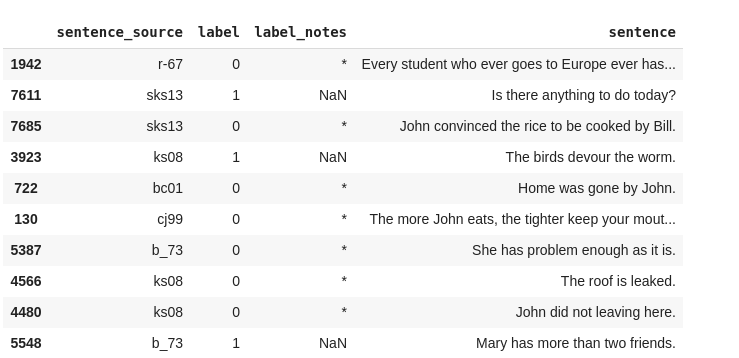we only need Sentences and labels , so we are going to drop others.

``````data.drop(['sentence_sources','label_note'],axis=1,inplace=True)
sentences=data.sentence.values
labels = data.label.values
``````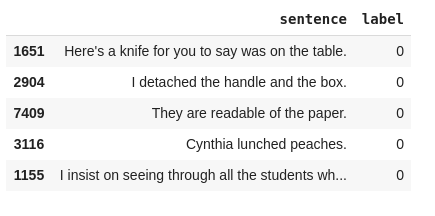###### Transforming the Dataset

Next step to getting BERT tokenizer as we have to split the sentences into token and mapped these token to the BERT tokenizer vocabulary to feed into the BERT model.

``````from transformers import BertTokenizer
# using the low level BERT for our task.
tokenizer = BertTokenizer.from_pretrained('bert-base-uncased', do_lower_case=True)

# Printing the original sentence.
print(' Original: ', sentences)

# Printing the tokenized sentence in form of list.
print('Tokenized: ', tokenizer.tokenize(sentences))

# Print the sentence mapped to token ids.
print('Token IDs: ', tokenizer.convert_tokens_to_ids(tokenizer.tokenize(sentences)))
``````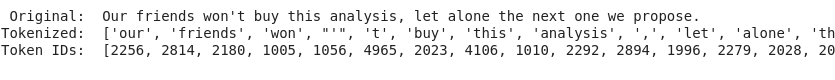###### Converting the input into suitable format for feeding into the bert

So actually BERT have it's requirement the input must be in a specific form which we are going discuss.
This specific input format have following part:

Special tokens
The input should be start with token known as 'CLS' and ending token must be 'SEP' token ,the tokenizer values for these token are 101 and 102 respectively.So we have to prepend 'CLS' and append 'SEP' tokens to every sentences.

It looks like after performing these operations.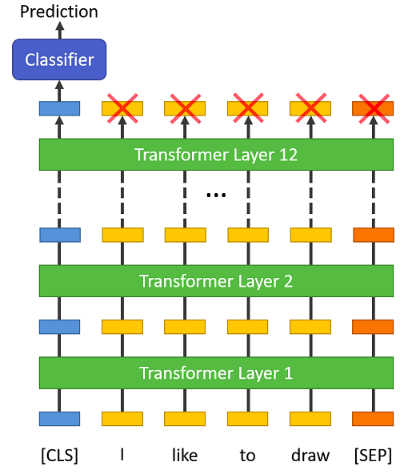We also have to specify the max length which we are going use , generally BERT all models support max_len upto 512(means apporx 512 words and exactly 512 tokens) but if our dataset don't have that lengthy sentences we can for smaller.we are going for MAX_len = 128 , it lesser time to train a model with less max_len as you can see.

Combining all the above steps by tokenizer.encode,

``````input_ids = []
for sent in sentences:
# so basically encode tokenizing , mapping sentences to thier token ids after adding special tokens.
encoded_sent = tokenizer.encode(
sent,                      # Sentence which are encoding.

)

input_ids.append(encoded_sent)
``````

We are going to pad the smaller ecoded sentences and truncate the larger encoded sentences to a fixed len (max_len).

``````from keras.preprocessing.sequence import pad_sequences

MAX_LEN = 128

``````

So attention masks help the model to recognize between actual words encoding and padding.

``````attention_masks = []

for sent in input_ids:

# Generating attention mask for sentences.
#   - when there is 0 present as token id we are going to set mask as 0.
#   - we are going to set mask 1 for all non-zero positive input id.
att_mask = [int(token_id > 0) for token_id in sent]

``````

Finally we able to transform the data into required format .

#### Training model

First we have to split the dataset into training and testing.

Training & Validation Split

``````from sklearn.model_selection import train_test_split

train_inputs, validation_inputs, train_labels, validation_labels = train_test_split(input_ids, labels, test_size=0.2)
``````

Utilizing the GPU

``````#changing the numpy arrays into tensors for working on GPU.
train_inputs = torch.tensor(train_inputs)
validation_inputs = torch.tensor(validation_inputs)

train_labels = torch.tensor(train_labels)
validation_labels = torch.tensor(validation_labels)

from torch.utils.data import TensorDataset, DataLoader, RandomSampler, SequentialSampler

# Deciding the batch size for training.

batch_size = 32

train_sampler = RandomSampler(train_data)

# DataLoader for our validation(test) set.
validation_sampler = SequentialSampler(validation_data)
``````

Getting the pre-trained BERT(base uncased with 12 layers)model, instead of going for original BERT we are going for it's variation for specific task (for text classification).

``````from transformers import BertForSequenceClassification, AdamW, BertConfig

model = BertForSequenceClassification.from_pretrained(
"bert-base-uncased",
num_labels = 2,
output_attentions = False,
output_hidden_states = False,
)

# Running the model on GPU.
model.cuda()
``````

It's should look something like this.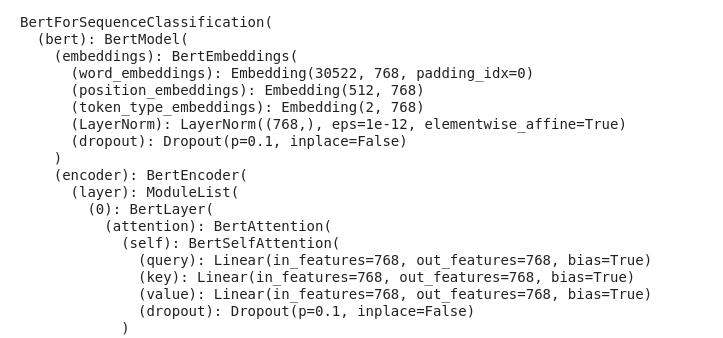###### Optimizer

Authors gone for AdamW optimizers instead of usual one SGD you can it in paper why they did that.

``````optimizer = AdamW(model.parameters(),
lr = 2e-5,
eps = 1e-8
)
``````

The code is too big i'm focusing in more important parts you can find full code here

###### Training

I have only important steps in training section you can follow above link to get full code.

``````loss_values = []

for epoch_i in range(0, epochs):
t0 = time.time()

total_loss = 0
# putting model in traing mode there are two model eval and train for model
model.train()

#checking time taken after every 50 steps.
if step % 50 == 0 and not step == 0:
elapsed = format_time(time.time() - t0)

b_input_ids = batch.to(device)
b_labels = batch.to(device)

outputs = model(b_input_ids,
token_type_ids=None,
labels=b_labels)

loss = outputs

total_loss += loss.item()

# doing back propagation
loss.backward()

optimizer.step()

# Update the learning rate.
scheduler.step()

``````

As you can see it's take a lot of time even in fine tuning as compared to other models.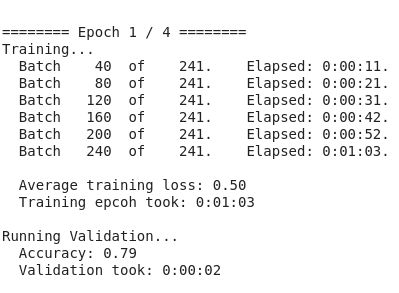#### Endnote

Here we have discussed only one application of BERT in future blog we try to cover as many application as we can .I think it seems to clear here that how bert can be used to achieve better result than others model out there.#### Aryanshu Verma

Student at Indian Institute of Information Technology (IIIT), Gwalior | Intern at OpenGenus

Application of BERT : Binary Text Classification